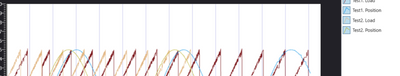# Measurement Studio for .NET Languages

cancel
Showing results for
Did you mean:

## WPF Legend Visibility Issue

Hi All,

I have an application where I have to plot data based on user selection and show them in a legend. So I have to create plots dynamically and add plot labels from a ViewModelThen I have binded Legend to these plots and got the labels displayed on legend.The problem is - clicking on the legend items no longer change the visibility of the plots.

Thanks,

Message 1 of 2
(333 Views)

## Re: WPF Legend Visibility Issue

Unfortunately, I could not reproduce the issue based on the code snippets you showed. Here is the XAML I used to define the graph and legend in my test project:

``````<Grid>
<Grid.ColumnDefinitions>
<ColumnDefinition Width="3*" />
<ColumnDefinition Width="*" />
</Grid.ColumnDefinitions>

<ni:Graph x:Name="AnalysisGraph" />

<ni:Legend Grid.Column="1" ItemsSource="{Binding ElementName=AnalysisGraph, Path=AllPlots}" />
</Grid>``````

And the code used to populate it with random data:

``````public MainWindow( ) {
InitializeComponent( );

var random = new Random( );
for( int i = 0; i < 2; i += 1 ) {
double offset = i * 2;
double[] load = GetData( random, offset - 0.5 );
double[] position = GetData( random, offset + 0.5 );
string name = "Test" + (i + 1);

AnalysisGraph.Plots.Add( new Plot( name + ". Load" ) );
AnalysisGraph.Data.Add( new List<object>( ) { load } );
AnalysisGraph.Plots.Add( new Plot( name + ". Position" ) );
AnalysisGraph.Data.Add( new List<object>( ) { position } );
}
}``````

Clicking on the legend glyphs shows and hides the corresponding plot like normal (see "Test2. Load"):You may want to try using WPF Snoop to debug if there is a binding problem with the legend glyph.

~ Paul H
Message 2 of 2
(312 Views)